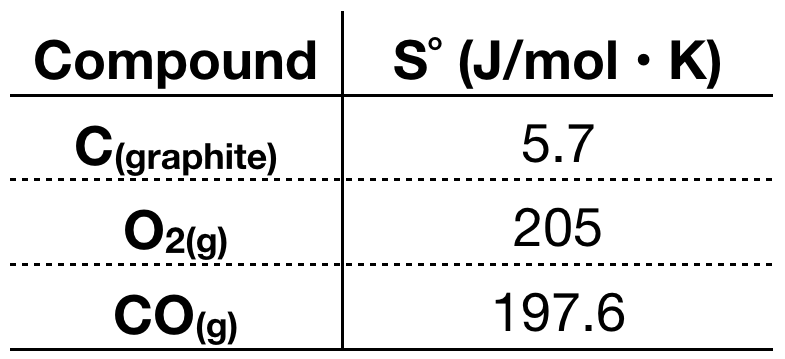Chemistry Practice Problems Entropy Practice Problems Solution: Consider the following reaction at 298 K:2C (graph...

🤓 Based on our data, we think this question is relevant for Professor Grant's class at HUNTER.

# Solution: Consider the following reaction at 298 K:2C (graphite) + O2 (g) → 2CO (g)  Delta H = -110.5 kJCalculate:Delta S(sys) J/KDelta S (surr) J/KDelta S (Univ) J/K

###### Problem

Consider the following reaction at 298 K:

2C (graphite) + O2 (g) → 2CO (g)  Delta H = -110.5 kJ

Calculate:

Delta S(sys) J/K
Delta S (surr) J/K
Delta S (Univ) J/K

###### Solution

#1) To calculate for ΔSsys, we need the S˚ of each compound in the reaction.View Complete Written Solution

Entropy

Entropy

#### Q. Consider the following reaction at 298 K2Al (s) + 3Cl2 (g) → 2AlCl3 (s)         H=-1408 KJCalculate the following quantities:(a) Ssys= ? (b) Ssurr= ?(...

Solved • Wed Jan 03 2018 14:34:48 GMT-0500 (EST)

Entropy

#### Q. Which of the following reactions have a positive ΔSrxn? Check all that apply.A. A(g) + B(g) → 3 C(g)B. A(g) + B(s) → C(g)C. A(g) + B(g) → C(g)D. A(g) ...

Solved • Fri Dec 15 2017 16:37:06 GMT-0500 (EST)

Entropy

#### Q. Which of the following reactions have a positive ΔS rxn?  a) 2 A(g) + B(s) → 3 C(g) b) 2 A(g) + B(g) → C(g) c) A(g) + B(g) → C(g) d) 2 A(g) + 2 B(...

Solved • Fri Dec 15 2017 16:34:49 GMT-0500 (EST)

Entropy

#### Q. A favorable entropy change occurs when ΔS is positive. What can be said about the order of the system when ΔS is positive.a) system order increasesb) ...

Solved • Thu Dec 14 2017 12:50:18 GMT-0500 (EST)